# String Basic

string ：有排序的字元集合

substring：一段連續的字串子集合

subsequence：依斷按順序但可不連續的字串子集合

suffix：從後面開始一樣的子字串

prefix：從前面開始一樣的子字串

ex:

String = abcdefg

Substring = “abcd”, “efg”… (連續)

Subsequence = “acdf”, “dfg”…. (不連續)

suffix = “efg”, “defg”….

prefix = “abcd”, “ab”…

# Original

## 程式碼

for(int i=0; i+lenB<=lenA; ++i)
{
int mat=0;
while( mat<lenB && A[i+mat]==B[mat] ) ++mat;
if(mat == lenB) print(i);
}

• A = “abcdefg”
• B = “cde”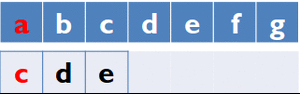worst case

• A = “aaaaa….aaa”

• B = “aaaaa….aab”# Hashing

• 要求：

1.函數f容易取得

1. 字串分佈均勻，意即碰撞次數愈小愈好
• 思考：
1. f(A) ≠ f(B) ，可以推得 A ≠ B

2. f(A) = f(B) ，可以推得 A = B or A ≠ B (發生碰撞)

3. 若分n類,碰撞機率為1/n

# Rabin-Karp rolling hash functionp,q取不同的質數可讓字串分佈的更加均勻

## 比對：rolling

1.先計算A所有prefix的hash value,時間複雜度為 O(|A|)

2.可以得出任何子字串的hash value

3.枚舉A長度為 |B| 的子字串,比較hash value,時間複雜度為O(n)

ex:

A = “abcdefg”

B = “cde”

# KMP

## Knuth-Morris-Pratt algorithm

ex:

B : aabaab

B字串本身重複 “aab”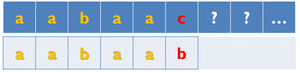## Fail FunctionB [ ] … 存放字串B

pi [ ] … 紀錄前一個重複字串出現的位置

cur_pos … 目前字串重複的位置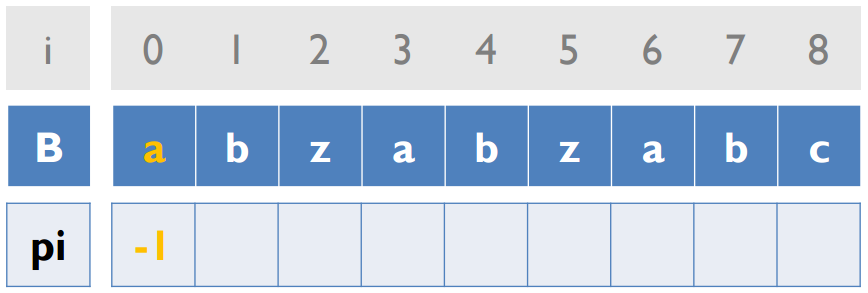cur_pos 初始為 -1 pi 初始為 -1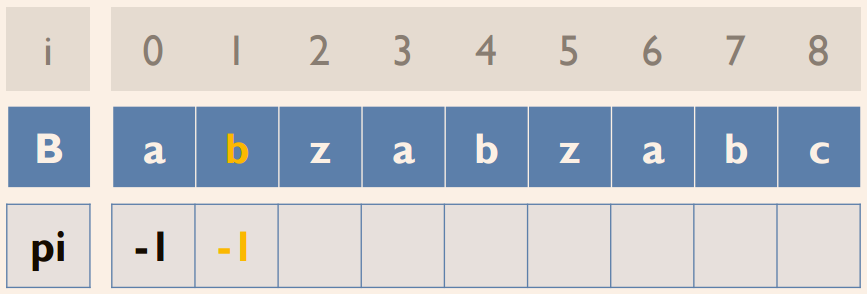B[cur_pos+1]!=B[i] pi[i]=cur_pos

void fail(string B, int *pi)
{
int len = strlen(B);
pi = -1;
for( int i = 1, cur_pos = -1; i<len; ++i)
{
while(cur_pos >= 0 && B[i] != B[cur_pos+1])
cur_pos = pi[cur_pos];
if(B[i] == B[cur_pos+1])  ++cur_pos;
pi[i] = cur_pos;
}
}

## Matching

Fail function: 找出各後綴與前綴一樣的最大值String Matching process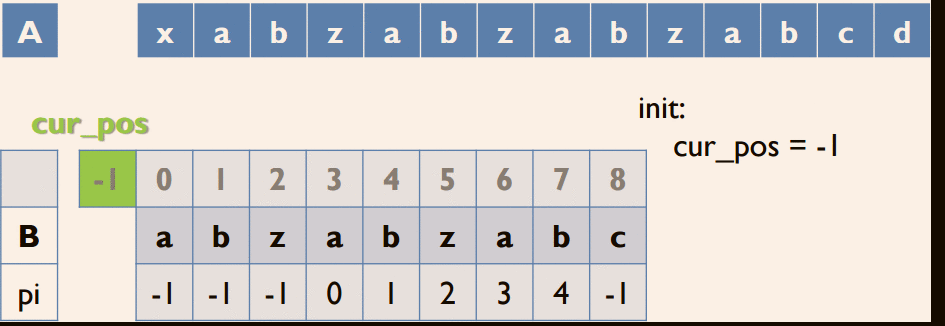Matching 與 Fail function 的作法類似，差別只在於比較的對象不同

void fail(string A, string B, int *pi)
{
int lenA = a.length();
int lenB = b.length();
for( int i = 0, cur_pos = -1; i<lenA; ++i)
{
while(cur_pos >= 0 && A[i] != B[cur_pos+1])
cur_pos = pi[cur_pos];
if(A[i] == B[cur_pos+1])  ++cur_pos;
if(cur_pos+1 == lenB)
{
/*Match!!!!!*/
cur_pos = pi[cur_pos];
}
}
}

Fail function + Matching • Complexity – 關鍵: while-loop – cur_pos 每次只會 +1 或往前 – 均攤後 𝒪( A + B )

# Z Algorithm

## Z_value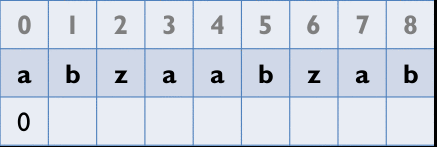## Z_Box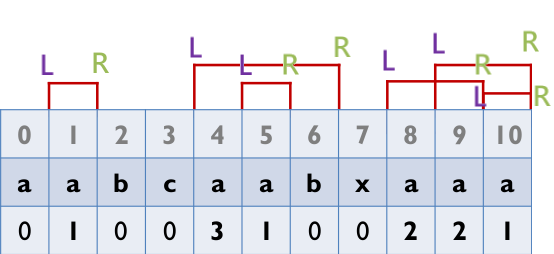## 如何算出Z_value?

• case 1: 自己沒有被別人的Z_Box包住 就乖乖數
•L在別人的Z_Box內 i’ 為 i 對應到前綴的位置 (像上面表格的aab(i=5)的a 對應到i=1 的a)
• case 2: 若自己的R在剛剛包含自己的Z_Box裏面 ( i’+z[i’] < z[L] => 沒有超過別人Z_Box的右界) 那麼z( i )=z( i’ )
•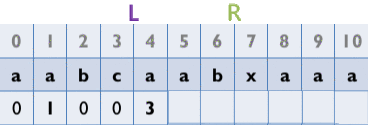Z_value=Z_value

• case 3: 若i’+z[i’] >= z[L]代表自己的Z_Box的右邊界和包住自己的Z_Box的右邊界重疊或超過
這時因為無法判斷在包住自己的Z_Box右邊界之後的element情形 所以Z_value只能算到包住自己的Z_Box的右邊界 之後的element都要去一個一個比對
•for a(i=9) its Z_value is 2 ,differ from Z_value

## Code## 使用方法

當要看B字串是不是A的子字串時，用一個沒有出現過的符號放在兩者之間， 並用Z algorithm，若A字串內的字元有Z_value等於B的長度，即B出現在A裡面A = “aabcc” B=“aab” 寫成B $A => aab$ aabcc